Next: Information dimension Up: Correlation dimension Previous: Takens-Theiler estimator

### Gaussian kernel correlation integral

The correlation sum Eq.() can be regarded as an average density of points where the local density is obtained by a kernel estimator with a step kernel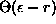. A natural modification for small point sets is to replace the sharp step kernel by a smooth kernel function of bandwidth. A particularly attractive case that has been studied in the literature  is given by the Gaussian kernel, that is,is replaced by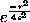. The resulting Gaussian kernel correlation sum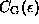has the same scaling properties as the usual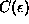. It has been observed in  thatcan be obtained fromvia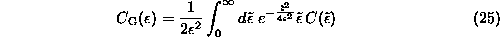without having to repeat the whole computation. Ifis given at discrete values of, the integrals in Eq.() can be carried out numerically by interpolatingwith pure power laws . This is done in c2g which uses a 15 point Gauss-Kronrod rule for the numerical integration.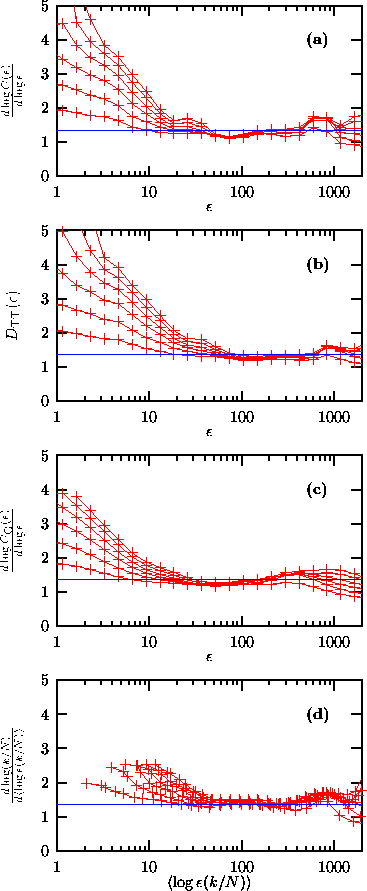Figure:   Dimension estimation for the (noise filtered) NMR laser data. Embedding dimensions 2 to 7 are shown. From above: (a) slopes are determined by straight line fits to the log-log plot of the correlation sum, Eq. (). (b) Takes-Theiler estimator of the same slope. (c) Slopes are obtained by straight line fits to the Gaussian kernel correlation sum, Eq.(). (d) Instead of the correlation dimension, it has been attempted to estimate the information dimension.Next: Information dimension Up: Correlation dimension Previous: Takens-Theiler estimator

Thomas Schreiber
Wed Jan 6 15:38:27 CET 1999